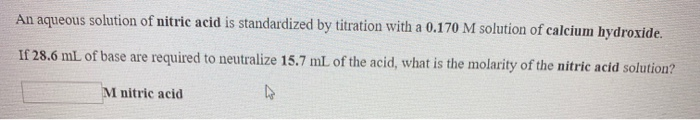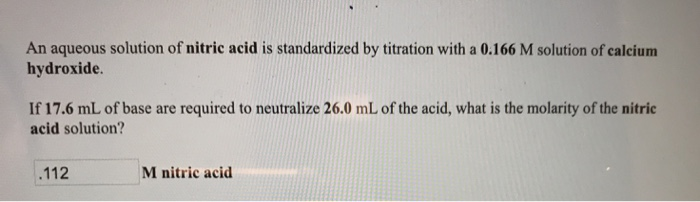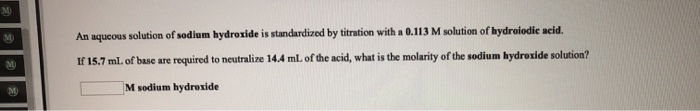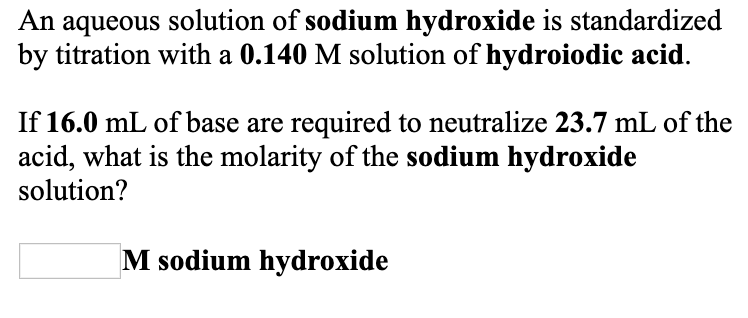Question

# An aqueous solution of nitric acid is standardized by titration with a 0.164 M solution of...

An aqueous solution of nitric acid is standardized by titration with a 0.164 M solution of barium hydroxide.

If 11.1 mL of base are required to neutralize 28.5 mL of the acid, what is the molarity of the nitric acid solution?

______M nitric acid

We need at least 10 more requests to produce the answer.

0 / 10 have requested this problem solution

The more requests, the faster the answer.

All students who have requested the answer will be notified once they are available.

#### Earn Coins

Coins can be redeemed for fabulous gifts.

Similar Homework Help Questions
• ### An aqueous solution of hydroiodic acid is standardized by titration with a 0.164 M solution of...

An aqueous solution of hydroiodic acid is standardized by titration with a 0.164 M solution of calcium hydroxide. If 27.3 mL of base are required to neutralize 20.0 mL of the acid, what is the molarity of the hydroiodic acid solution? ______M hydroiodic acid

• ### An aqueous solution of barium hydroxide is standardized by titration with a 0.199 M solution of...

An aqueous solution of barium hydroxide is standardized by titration with a 0.199 M solution of hydroiodic acid. If 19.1 mL of base are required to neutralize 18.2 mL of the acid, what is the molarity of the barium hydroxide solution? ______M barium hydroxide

• ### An aqueous solution of nitric acid is standardized by titration with a 0.170 M solution of...An aqueous solution of nitric acid is standardized by titration with a 0.170 M solution of calcium hydroxide. If 28.6 mL of base are required to neutralize 15.7 mL of the acid, what is the molarity of the nitric acid solution? M nitric acid

• ### An aqueous solution of hydroiodic acid is standardized by titration with a 0.123 M solution of...

An aqueous solution of hydroiodic acid is standardized by titration with a 0.123 M solution of calcium hydroxide. If 10.7 mL of base are required to neutralize 10.9 mL of the acid, what is the molarity of the hydroiodic acid solution? ____M hydroiodic acid An aqueous solution of barium hydroxide is standardized by titration with a 0.114 M solution of hydrobromic acid. If 27.6 mL of base are required to neutralize 27.9 mL of the acid, what is the molarity...

• ### An aqueous solution of nitric acid is standardized by titration with a 0.166 M solution of calcium hydroxide If 17....An aqueous solution of nitric acid is standardized by titration with a 0.166 M solution of calcium hydroxide If 17.6 mL of base are required to neutralize 26.0 mL of the acid, what is the molarity of the nitric acid solution? M nitric acid .112

• ### An aqueous solution of sodium hydroxide is standardized by titration with a 0.113 M solution of...An aqueous solution of sodium hydroxide is standardized by titration with a 0.113 M solution of hydrolodic acid. If 16.7 ml. of base are required to neutralize 14.4 ml of the acid, what is the molarity of the sodium hydroxide solution? M sodium hydroxide An aqueous solution of barium hydroxide is standardized by titration with a 0.191 M solution of hydrolodic acid. If 19.1 ml of base are required to neutralize 25.9 mL of the acid, what is the molarity...

• ### An aqueous solution of sodium hydroxide is standardized by titration with a 0.140 M solution of...An aqueous solution of sodium hydroxide is standardized by titration with a 0.140 M solution of hydroiodic acid. If 16.0 mL of base are required to neutralize 23.7 mL of the acid, what is the molarity of the sodium hydroxide solution? M sodium hydroxide An aqueous solution of hydrobromic acid is standardized by titration with a 0.106 M solution of barium hydroxide. If 25.8 mL of base are required to neutralize 29.4 mL of the acid, what is the molarity...

• ### An aqueous solution of hydrobromic acid is standardized by titration with a 0.165 M solution of...

An aqueous solution of hydrobromic acid is standardized by titration with a 0.165 M solution of barium hydroxide. If 19.0 mL of base are required to neutralize 22.9 mL of the acid, what is the molarity of the hydrobromic acid solution?

• ### An aqueous solution of hydrobromic acid is standardized by titration with a 0.121 M solution of...

An aqueous solution of hydrobromic acid is standardized by titration with a 0.121 M solution of barium hydroxide. If 12.9 mL of base are required to neutralize 13.6 mL of the acid, what is the molarity of the hydrobromic acid solution?

• ### An aqueous solution of hydrobromic acid is standardized by titration with a 0.172 M solution of...

An aqueous solution of hydrobromic acid is standardized by titration with a 0.172 M solution of barium hydroxide. If 29.7 mL of base are required to neutralize 23.3 mL of the acid, what is the molarity of the hydrobromic acid solution?